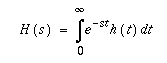# HTML+ Mathematical Equation Support

A whole collection of SGML tags for mathematical equations are also proposed for HTML+. The MATH tag is used to markup a mathematical formula. Tags such as SUP, SUB, BOX, ARRAY and a set of character entities are defined. HTML math support is similar to TeX.

Here is an example:

```[itex]
H(s) = ∫<sub>0</sub><sup>∞</sup> e<sup>-st</sup> h(t) dt
[/itex]
```

Here is how this might be rendered: